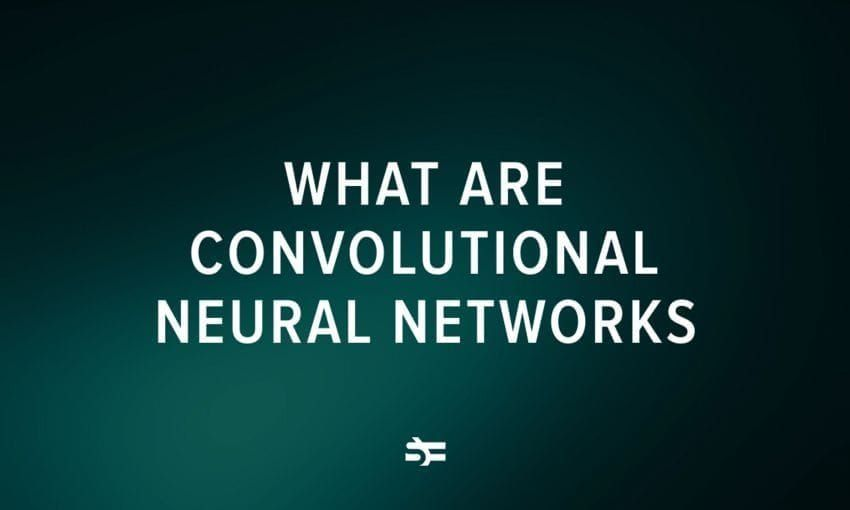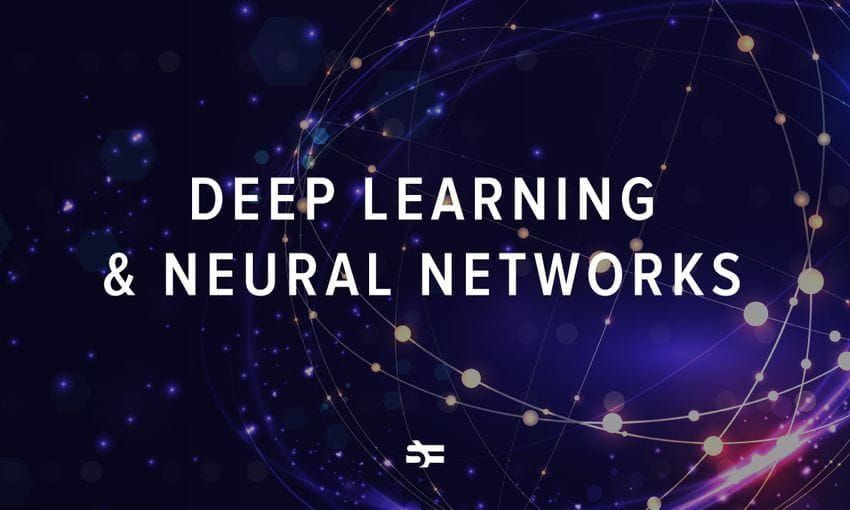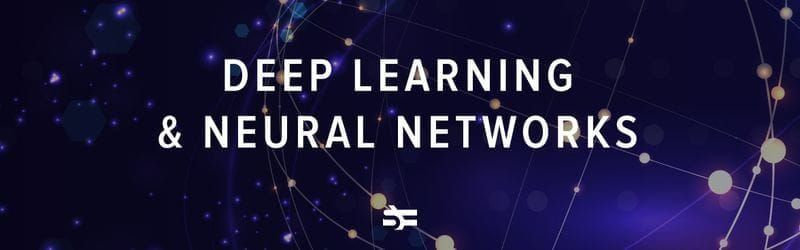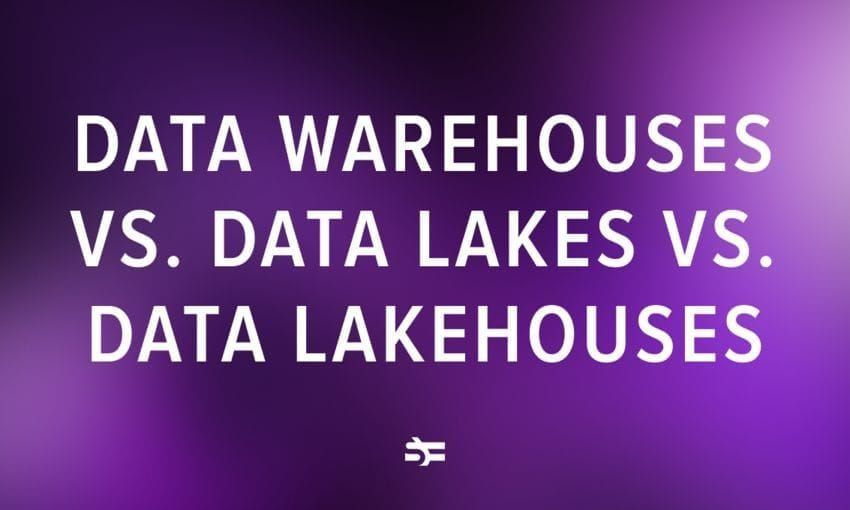# An Overview of Machine Learning Optimization Techniques

The principal goal of machine learning is to create a model that performs well and gives accurate predictions in a particular set of cases. In order to achieve that, we need machine learning optimization.

Machine learning optimization is the process of adjusting hyperparameters in order to minimize the cost function by using one of the optimization techniques. It is important to minimize the cost function because it describes the discrepancy between the true value of the estimated parameter and what the model has predicted.

Serokell’s team has extensive experience in ML project development, and in this post, we will tell you about the main types of ML optimization techniques.

## Parameters and hyperparameters of the model

Before we go any further, we need to understand the difference between parameters and hyperparameters of a model. These two notions are easy to confuse but we ought not to.

• You need to set hyperparameters before starting to train the model. They include a number of clusters, learning rate, etc. Hyperparameters describe the structure of the model.
• On the other hand, the parameters of the model are obtained during the training. There is no way to get them in advance. Examples are weights and biases for neural networks. This data is internal to the model and changes based on the inputs.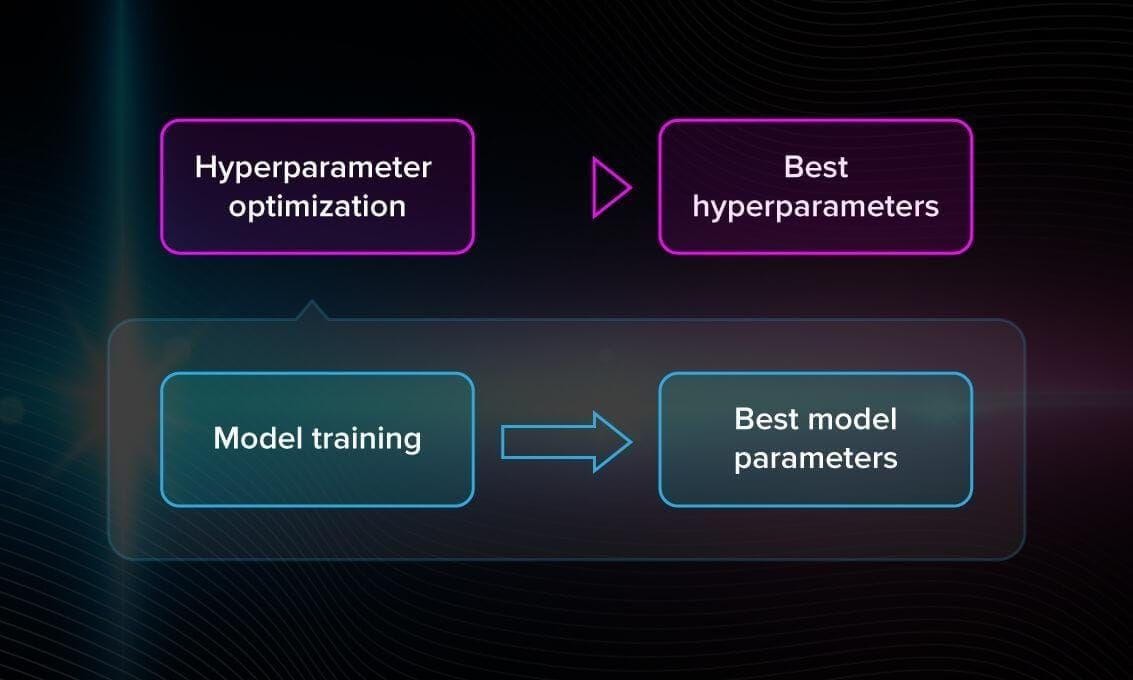To tune the model, we need hyperparameter optimization. By finding the optimal combination of their values, we can decrease the error and build the most accurate model.

## How hyperparameter tuning works

As we said, the hyperparameters are set before training. But you can’t know in advance, for instance, which learning rate (large or small) is best in this or that case. Therefore, to improve the model’s performance, hyperparameters have to be optimized.

After each iteration, you compare the output with expected results, assess the accuracy, and adjust the hyperparameters if necessary. This is a repeated process. You can do that manually or use one of the many optimization techniques, which come in handy when you work with large amounts of data.

## Top optimization techniques in machine learning

Now let us talk about the techniques that you can use to optimize the hyperparameters of your model.

Exhaustive search, or brute-force search, is the process of looking for the most optimal hyperparameters by checking whether each candidate is a good match. You perform the same thing when you forget the code for your bike’s lock and try out all the possible options. In machine learning, we do the same thing but the number of options is quite large, usually.

The exhaustive search method is simple. For example, if you are working with a k-means algorithm, you will manually search for the right number of clusters. However, if there are hundreds and thousands of options that you have to consider, it becomes unbearably heavy and slow. This makes brute-force search inefficient in the majority of real-life cases.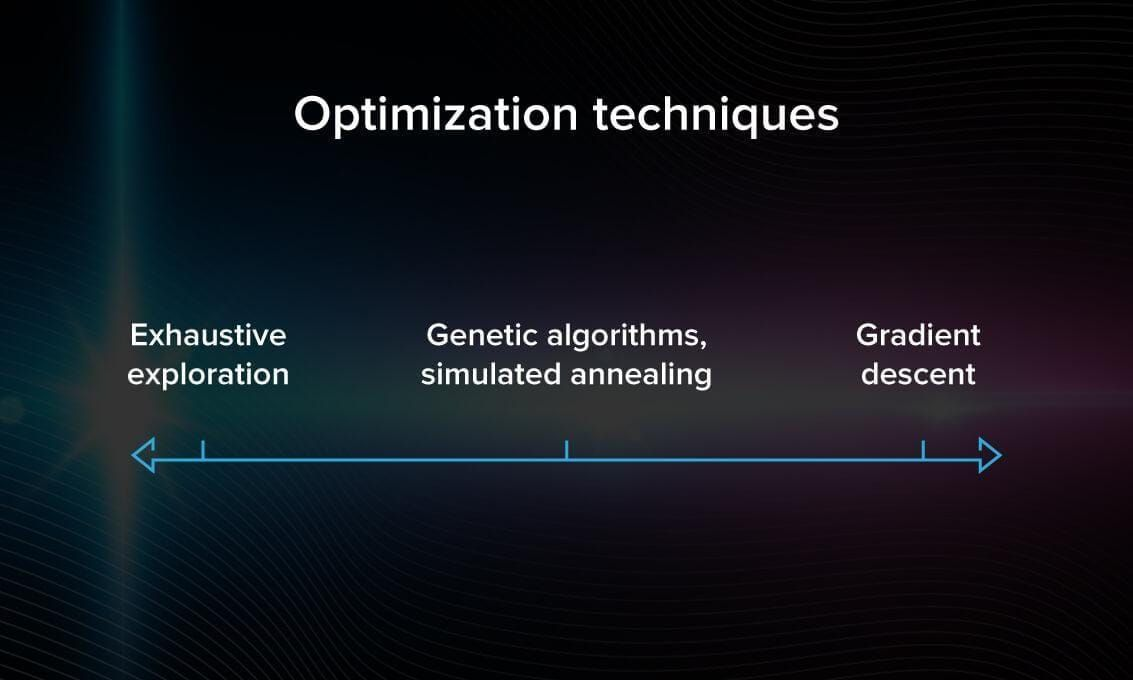Gradient descent is the most common algorithm for model optimization for minimizing the error. In order to perform gradient descent, you have to iterate over the training dataset while re-adjusting the model.

Your goal is to minimize the cost function because it means you get the smallest possible error and improve the accuracy of the model.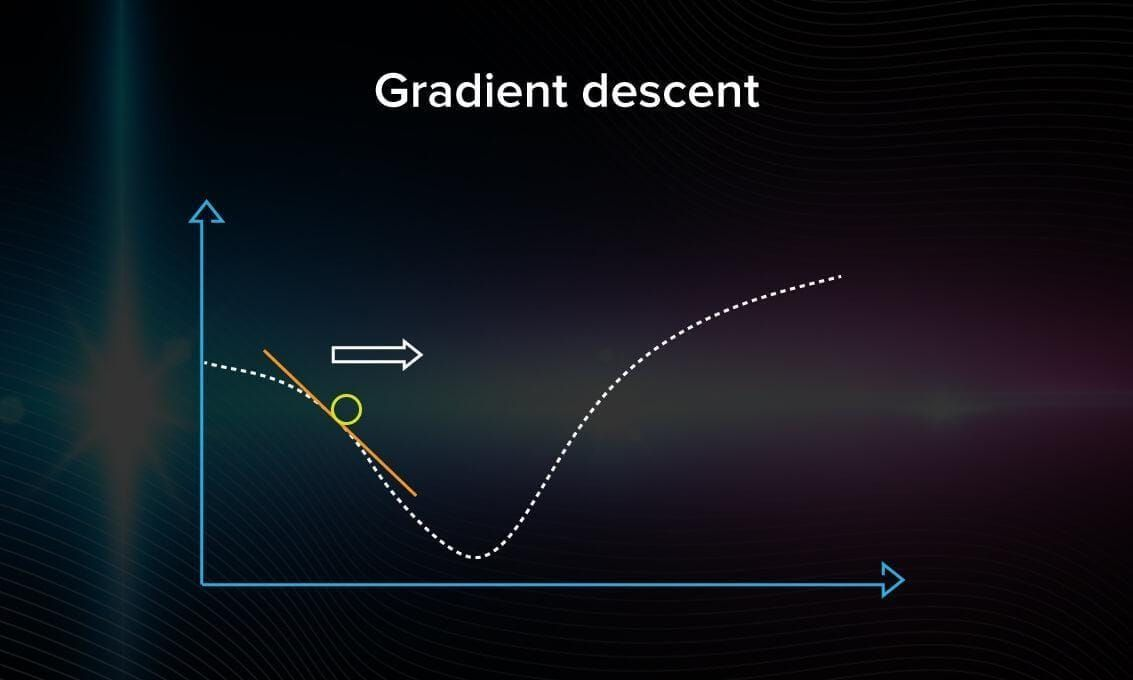On the graph, you can see a graphical representation of how the gradient descent algorithm travels in the variable space. To get started, you need to take a random point on the graph and arbitrarily choose a direction. If you see that the error is getting larger, that means you chose the wrong direction.

When you are not able to improve (decrease the error) anymore, the optimization is over and you have found a local minimum. In the following video, you will find a step-by-step explanation of how gradient descent works.

Looks fine so far. However, classical gradient descent will not work well when there are a couple of local minimums. Finding your first minimum, you will simply stop searching because the algorithm only finds a local one, it is not made to find the global one.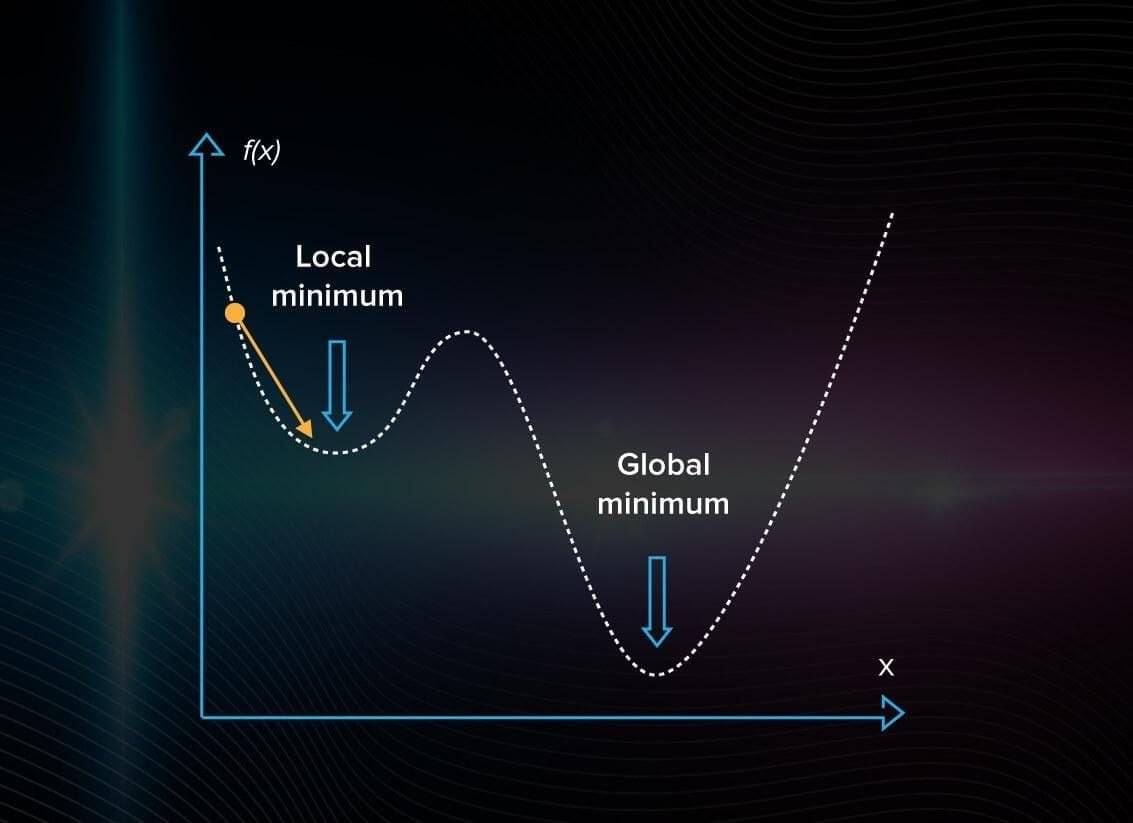Note: In gradient descent, you proceed forward with steps of the same size. If you choose a learning rate that is too large, the algorithm will be jumping around without getting closer to the right answer. If it’s too small, the computation will start mimicking exhaustive search take, which is, of course, inefficient.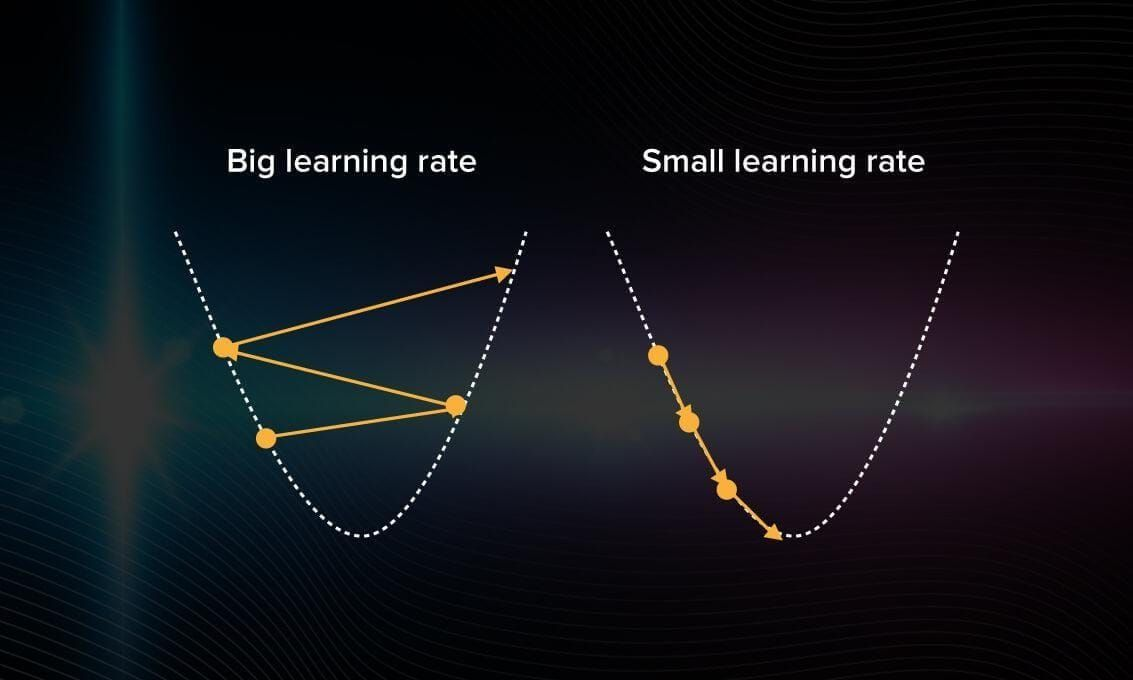So you have to choose the learning rate very carefully. If done right, gradient descent becomes a computation-efficient and rather quick method to optimize models.

### Genetic algorithms

Genetic algorithms represent another approach to ML optimization. The principle that lays behind the logic of these algorithms is an attempt to apply the theory of evolution to machine learning.

In the evolution theory, only those specimens get to survive and reproduce that have the best adaptation mechanisms. How do you know what specimens are and aren’t the best in the case of machine learning models?

Imagine you have a bunch of random algorithms at hand. This will be your population. Among multiple models with some predefined hyperparameters, some are better adjusted than the others. Let’s find them! First, you calculate the accuracy of each model. Then, you keep only those that worked out best. Now you can generate some descendants with similar hyperparameters to the best models to get a second generation of models.

You can see the logic behind this algorithm in this picture: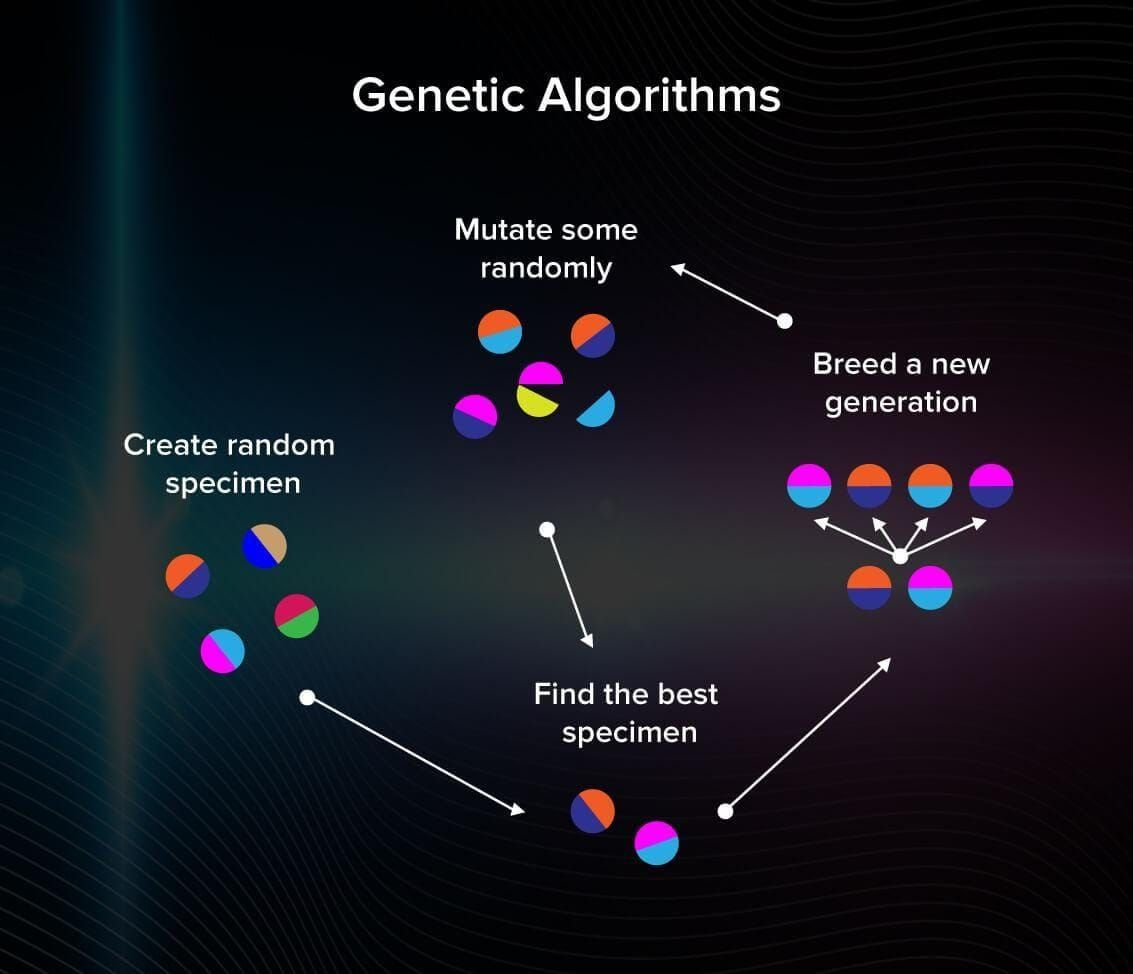We repeat this process many times and only the best models will survive at the end of the process. Genetic algorithms help to avoid being stuck at local minima/maxima. They are common in optimizing neural network models.

Algorithm Pros Cons Where to use
Exhaustive search
• All possible options are evaluated.
• The most intuitive one.
• When there are many solutions, it becomes extremely slow.
• The database is small.
• High accuracy is more important than the cost and speed of comp.
• Computation efficient.
• Stable.
• Easy and quick to use.
• Doesn't work if there are multiple local minima.
• If the learning rate is too large, you risk skipping the right solution.
• Need to optimize the model fast.
• You can’t calculate the parameters linearly and have to search for them.
Genetic
• Can find good solutions in a short computation time.
• Wide range of solutions (since it’s random).
• Can’t guarantee that the solution is optimal.
• Hard to come up with good heuristics.
• Need to avoid getting stuck in local minima.

## Optimization of deep learning models

It is important to use good, cutting-edge algorithms for deep learning instead of generic ones since training takes so much computing power.

Stochastic gradient descent with momentum, RMSProp, and Adam Optimizer are algorithms that are created specifically for deep learning optimization. There is a series of videos about neural network optimization that covers these algorithms on deeplearning.ai, which we recommend viewing.

### Stochastic gradient descent with momentum

The disadvantage of this method is that it requires a lot of updates, the steps of gradient descent are noisy. Because of this, the gradient can go in the wrong direction and become very computationally expensive. That is why other optimization algorithms are often used.

### RMSProp

RMSProp is useful to normalize the gradient itself because it balances out the step size. It can work even with the smallest batches.

Adam Optimizer can handle the noize problem and works even with large datasets and parameters.

It is hard and almost morally wrong to give general advice on how to optimize every ML model. That is why it is better to learn by example:

• If you’re interested in optimizing neural networks and comparing the effectiveness of different optimizers, give a try to Sanket Doshi’s post.
• You can also study how to optimize models with reinforcement learning with Berkey AI research.